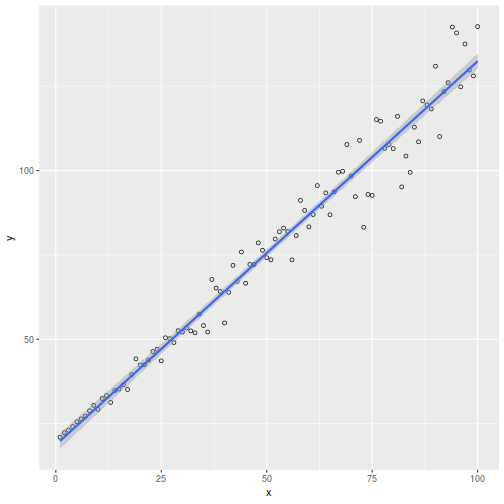# Is the pain worth it?: Can Rcpp speed up Passing Bablok Regression?

September 9, 2019
By

Want to share your content on R-bloggers? click here if you have a blog, or here if you don't.

### Background

R dogma is that for loops are bad because they are slow but this is not the case in C++. I had never programmed a line of C++ as of last week but my beloved firstborn started university last week and is enrolled in a C++ intro course, so I thought I would try to learn some and see if it would speed up Passing Bablok regression.

### Passing Bablok Regression

As mentioned in the past, the field of Clinical Chemistry has a peculiar devotion to Passing Bablok regression… and hey, why not?

Here is the code for a minimal implementation of Passing Bablok regression as discussed in this paper. This is the scale-invariant version.

PB.reg <- function(x,y){
lx <- length(x)
l <- lx*(lx - 1)/2
k <- 0
S <- rep(NA, lx)
for (i in 1:(lx - 1)) {
for (j in (i + 1):lx) {
k <- k + 1
S[k] <- (y[i] - y[j])/(x[i] - x[j])
}
}
S.sort <- sort(S)
N <- length(S.sort)
neg <- length(subset(S.sort,S.sort < 0))
K <- floor(neg/2)
if (N %% 2 == 1) {
b <- S.sort[(N+1)/2+K]
} else {
b <- sqrt(S.sort[N / 2 + K]*S.sort[N / 2 + K + 1])
}
a <- median(y - b * x)
return(as.vector(c(a,b)))
}

So let’s make some fake data and see what we get:

set.seed(314)
x <- seq(1, 100, length.out = 100)
y <- 1.1* x + 20 + x*rnorm(100,0,0.10)
reg <- PB.reg(x,y)
round(reg,2)

##  18.90  1.14Just to sanity check, we can get the coefficients from the mcr() package.

library(mcr)
reg.mcr <- mcreg(x,y)
round([email protected],2)

##  18.90  1.14

Ok, looks good.

### Rcpp

The Rcpp package permits compiling and execution of C++ code from within R. This can be good for computationally intensive tasks. Here is my child-like attempt at recapitulation of the R script above.

#include
#include
#include
#include
#include
#include
#include "Rcpp.h"
using namespace std;
using namespace Rcpp;
// [[Rcpp::plugins("cpp11")]]
// [[Rcpp::export]]

vector  PB(vector x,vector y){
int lx=x.size();
int l=lx*(lx-1)/2;
int k=-1;
vector  S(l,numeric_limits::quiet_NaN());
vector  sortS;
vector  neg;
double b;
double a;
vector  aVec(x.size());
vector  coef(2);

// Calculate the components of the S Matrix
for(int i=0; i :: iterator it = S.begin(); it != S.end(); ++it){
if (!isnan(*it)) sortS.push_back((*it));
}

// Put all the negative values into a vector called neg
for(vector  :: iterator it = S.begin(); it != S.end(); ++it){
if ((*it)<0) neg.push_back((*it));
}

// Calculate N and K
int K=int(floor(float(neg.size()/2)));
int N=sortS.size();

// Calculate the slope
if (N%2==1) {
b=S[static_cast ((N+1)/2+K-1)];
} else {
b=sqrt(S[static_cast (N/2+K-1)]*S[static_cast (N/2+K)]);
}

// Make a vector aVec of the estimates of the intercept
for(int i=0; i((x.size()+1.0)/2.0-1.0)];
}else{
a=(aVec[static_cast (x.size()/2.0-1.0)] + aVec[static_cast (x.size()/2.0)])/2.0;
}

// Report results
coef=a;
coef=b;
return coef;
}

int main(){
return 0;
}

Saving this to a local directory as PB_Reg.cpp, we can compile it and call the function into the R session as follows:

library(Rcpp)
sourceCpp("PB_Reg.cpp")

And run it:

round(PB(x,y),2)

##  18.90  1.14

Looks good! But is it faster? Like really faster? Let’s find out. And let’s also compare it to what the compiler() package produces:

library(compiler)
PB.reg.cmpfun <- cmpfun(PB.reg)

library(rbenchmark)
bmark <- benchmark(
Rscript = PB.reg(x,y),
Compiler Package = PB.reg.cmpfun(x,y),
Rcpp = PB(x,y),
replications = 100,
order = "relative"
)
knitr::kable(bmark)

test replications elapsed relative user.self sys.self user.child sys.child
3 Rcpp 100 0.024 1.000 0.024 0.000 0 0
2 Compiler Package 100 0.214 8.917 0.214 0.000 0 0
1 Rscript 100 0.217 9.042 0.213 0.004 0 0

So this makes C++ code about 9x faster than R source code. Not bad but in this case it was probably not worth the effort. Oh well. I learned something. Incidentally, the compiler() package did not help much in this case as it is only marginally better than raw R source. I have seen better results from compiler() in other cases.

### Final thought

Speaking of speed:

“Free yourself, like a gazelle from the hand of the hunter, like a bird from the snare of the fowler.”

Proverbs 6:5

R-bloggers.com offers daily e-mail updates about R news and tutorials about learning R and many other topics. Click here if you're looking to post or find an R/data-science job.
Want to share your content on R-bloggers? click here if you have a blog, or here if you don't.